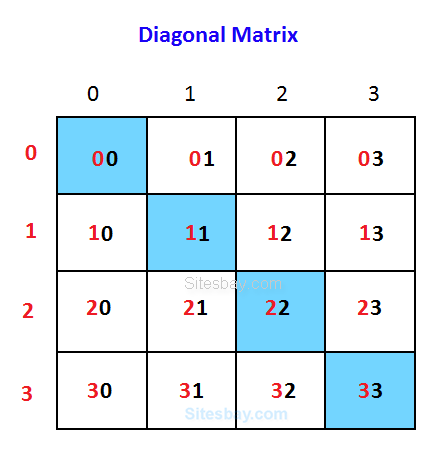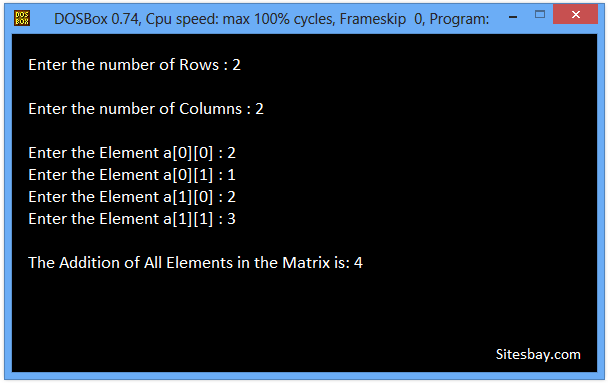# Find Sum of Diagonal Elements of Matrix Program in C

## C Program to Find Sum of Diagonal Elements of Matrix

Using this code we find the sum of diagonal elements of a square matrix.For example, for a 2 x 2 matrix, the sum of diagonal elements of the matrix {1,2,3,4} will be equal to 5.

To write this code is same as the sum of elements of a matrix, we add only those elements of the matrix for which row number and column number is same, like 1st row and 1st column, 2nd row and 2nd column and so on(i==j).

## Example

```1    2

3    4

Sum = 1+4 = 5
```

### Considering 3X3 matrix

• We have to add a,a,a
• By Observing, it is clear that when i = j Condition is true then and then only we have to add the elements## C Program to Find Sum of Diagonal Elements of Matrix

```#include<stdio.h>
#include<conio.h>

void main()
{

int i, j, matrix, row, col;
int sum = 0;
clrscr();

printf("\nEnter the number of Rows : ");
scanf("%d", &row);

printf("\nEnter the number of Columns : ");
scanf("%d", &col);

//Accept the Elements in m x n Matrix
for (i = 0; i < row; i++)
{
for (j = 0; j < col; j++)
{
printf("\nEnter the Element a[%d][%d] : ", i, j);
scanf("%d", &matrix[i][j]);
}
}

for (i = 0; i < row; i++)
{
for (j = 0; j < col; j++)
{
if (i == j)
sum = sum + matrix[i][j];
}
}

//Print out the Result
printf("\nSum of Diagonal Elements in Matrix is: %d", sum);
getch();
}
```

## Output

```Enter the number of Rows : 2

Enter the number of Columns : 2

Enter the Element a : 2
Enter the Element a : 1
Enter the Element a : 2
Enter the Element a : 3

The Addition of All Elements in the Matrix is: 4
```WIFI CCTV Camera